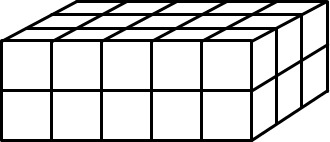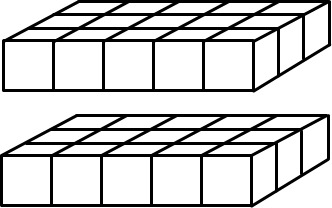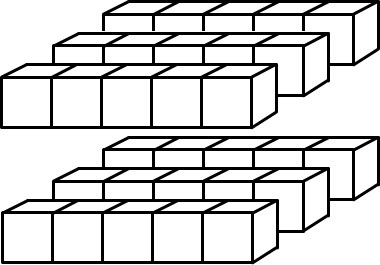# Using Volume to Understand the Associative Property of Multiplication

Alignments to Content Standards: 5.MD.C.5.a

Make sure you have plenty of snap cubes.

1. Build a rectangular prism that is 2 cubes on one side, 3 cubes on another, and 5 cubes on the third side.
2. We will say that the volume of one cube is 1 cubic unit. What is the volume of the rectangular prism?
3. Jenna said,
The rectangular prism is 2 cubes by 3 cubes by 5 cubes, so the volume of the prism is $2\times3\times5$ cubic units.
Ari said,
I don't know what $2\times3\times5$ means. Do you multiply the 2 and 3 first \begin{align} 2\times3\times5 &= (2\times3)\times5\\ &=6\times 5 \end{align} so you have 6 groups of 5, or do you multiply the 3 and the 5 first \begin{align}2\times3\times5 &= 2\times(3\times5)\\ &=2\times 15 \end{align} so you have 2 groups of 15?
• Explain how you can see the rectangular prism as being made of 2 groups with 15 cubes in each.
• Explain how you can also see the rectangular prism as being made of 6 groups with 5 cubes in each.
4. Does it matter which numbers you multiply first when you want to find the volume of a rectangular prism?

## IM Commentary

The purpose of this task is for students to use the volume of a rectangular prism to understand the associative property of multiplication. Note that students do not need to use the term "associative property" themselves, but they do need to understand that when you multiply three numbers, you can group them any way you want and still get the same result.

Note that 5.MD.C.5 states that students need to apply the formula $V=l\times w\times h$ to find the volume of a right rectangular prism. In fact, an expression like $2\times3\times5$ only makes sense because multiplication is associative. In other words, students must be comfortable with the idea that you can group the three factors in any way you wish and still get the same product in order to make sense of and apply this formula.

## Solution

1. A rectangular prism that is 2 cubes on one side, 3 cubes on another, and 5 cubes on the third side might look like this:2. The volume of the rectangular prism is 30 cubic units.
• If we cut the prism into two layers:we can see it as being made of 2 groups with 15 cubes in each.

• If we cut the prism into two layers and then cut each layer into three slices:we can see the prism as being made up of 6 slices with 5 cubes in each.

3. It doesn't matter which numbers you multiply first when you want to find the volume of a rectangular prism. We can see this most easily when the side-lengths are whole-numbers, because we can always cut the prism into layers (and then each layer into slices) to show the same phenomenon that we saw here. We can write this in symbols as follows: \begin{align} V&=l \times w \times h\\ &= l \times (w \times h)\\ &= (l \times w) \times h \end{align}